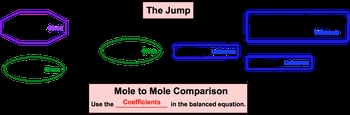Start typing, then use the up and down arrows to select an option from the list.# GOB Chemistry

Learn the toughest concepts covered in your GOB - General, Organic, and Biological Chemistry class with step-by-step video tutorials and practice problems.

Solutions

# Solution Stoichiometry

Solution Stoichiometry deals with stoichiometric calculations in solutions that involve volume and molarity.

## Solution Stoichiometry

1

#### concept

Solution Stoichiometry3m
Play a video:
solutions to it. Geometry deals with stock geometric calculations in solutions that involve volume and polarity. So if you've watched my videos on stock geometry, you are pretty familiar with our stock geometric chart. Now that we are including solutions toy geometry, we're gonna adapt it slightly to fit. The situations were now volume and polarity are sometimes given. So here, in our solutions Tokyo Metric chart. We're going to say the chart uses the given quantity of a compound to determine the unknown quantity of another compound. So for here in this example, we have our balanced chemical equation. And it says, for every two moles of sodium solid reacting with two moles off water as a liquid, we produce one mole of hydrogen gas and two moles of sodium hydroxide as an acquis product. Now they're giving to us that water has a volume of 38.74 mL off 0.275 Moeller. So this is aren't given information, and then what they're asking us to do is to determine the grams of H two. Now, remember, in our store geometric chart on the left side is where we have our given information. We are cut we're used to seeing are given information given to us in grands or in moles. But now it's solution. Story Geometry will tend to see it also be given to us as volume off Some more clarity. Remember, we've said this before The word off in between two numbers means multiply and realize here that moles equals leaders times more clarity. So if you were to change these MLS toe leaders and multiply them by the mole aren t Then you would see that this new given shape that we are seeing feeds directly into our moles of given And remember once we have our moles have given it's our job to get to our unknown are unknown is what we're looking to find here are unknown is h two and we need to find it in grams So following the story geometric chart we have here we go from the moles of given which is H 20 and we do the jump in the jump We're going from an area where we have information we know to an area where we don't know anything about the unknown And in this jump we have to remember to do a multiple comparison where we use the coefficients in the balanced equation. At this point, it would take us two moles of H two and then it's up to us to go to either grams of H two or ions. Adams formula units or molecules of whatever unknown would be. So again, this is a continuation of our idea with Stoke geometry. But now we're including this new shape here. If you haven't watched my video on Stoke geometry, I highly suggest you go back and take a look at the cup of those videos. It's just a continuation of that idea now, including polarity and volume within our calculations. Now that we've seen this new start geometric chart, let's move on to some questions where we put it to practice.

Use the solution stoichiometric chart when dealing with calculations involving molarity and/or volume.2

#### example

Solution Stoichiometry Example 13m
Play a video:
3
Problem

How many milliliters of 0.325 M HCl are needed to react with 16.2 g of magnesium metal?

2 HCl (aq) + Mg (s) → MgCl2 + H2 (g)

4
Problem

What is the molar concentration of a hydrobromic acid solution if it takes 34.12 mL of HBr to completely neutralize 82.56 mL of 0.156 M Ca(OH)­2

2 HBr (aq) + Ca(OH)2 (aq)  → CaBr2 (aq) + 2 H2O (l)

5
Problem

Consider the following balanced chemical equation:

H2O+ 2 MnO4 + 3 SO32-  → 2 MnO2 + 3 SO42-+ 2 OH

How many grams of MnO2 (MW:86.94 g/mol) will be created when 25.0 mL of 0.120 M MnO4 (MW:118.90 g/mol) reacts with 32.0 mL of 0.140 M SO32- (MW:80.07 g/mol).